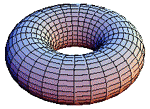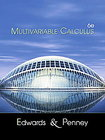Fall 2019
Multivariable Calculus
Math 18.02, Concourse - MIT
Fall 2019

Lectures and Recitations by:
Robert Winters
rwinters@mit.edu
Office: 16-137
Phone: x3-2050
(but e-mail is better)

 Calendar of topics and homework assignments Solutions

Concourse 18.02 Stellar siteThe text for the course is
Multivariable Calculus, 6th Edition by Edwards & Penney (ISBN 0130339679 for softcover edition)
[Click on the image below for prices.]• Ch. 12 - Vectors, Curves, and Surfaces in Space

• Ch. 13 - Partial Differentiation

• Ch. 14 - Multiple Integrals

• Ch. 15 - Vector Calculus

Announcements:

Thanks for attending the course. Course grades will be submitted shortly.
[Everyone who attended the course and took the Final Exam will be receiving a passing grade, so rest easy!]

 Approximate letter grades for Exam #1 Total points on exam was 50. Median score was 43.5. Mean score was 43.8. Standard deviation was 4.2. score grade score grade 45+ A 33+ C+ 43+ A– 30+ C 41+ B+ 27+ C– 39+ B 25+ D 36+ B– 0-24 F

 Approximate letter grades for Exam #2 Total points on exam was 50. Median score was 44. Mean score was 44.2. Standard deviation was 4.5. score grade score grade 45+ A 33+ C+ 43+ A– 30+ C 41+ B+ 27+ C– 39+ B 25+ D 36+ B– 0-24 F
 Approximate letter grades for Exam #3 Total points on exam was 50. Median score was 48. Mean score was 45.5. Standard deviation was 5.8. score grade score grade 45+ A 33+ C+ 43+ A– 30+ C 41+ B+ 27+ C– 39+ B 25+ D 36+ B– 0-24 F

 Approximate letter grades for Final Exam Total points on exam was 100. Median score was 84. Mean score was 80.7. Standard deviation was 10.7. score grade score grade 90+ A 65+ C+ 85+ A– 60+ C 80+ B+ 55+ C– 75+ B 50+ D 70+ B– 0-49 F

Exam #3 took place on Tuesday, Nov 19 during class. Topics included: Integration over two- and three-dimensional regions; double and triple integrals in Cartesian, cylindrical, and spherical coordinates; Fubini Theorem and interchanging order of integration; applications of integration – areas, volumes, mass, averaging, weighted averages, centroids and center of mass, moment of inertia, general change of variables for double and triple integrals, Jacobian determinants; Integration of functions over curves and surfaces (line and surface integrals) with applications to mass, averaging, centroids, flux, etc.; flux integrals in 2- and 3-dimensions; conservative vector fields and potential functions; Fundamental Theorem of Line Integrals, Green’s Theorem.

Exam #2 took place on Monday, Oct 28 during class. Topics included: Partial derivatives, linear approximation, differentials, gradient vector, normal vectors to curves and surfaces, directional derivative, the Chain Rule, implicit differentiation, stationary points, second derivative test, optimization, Method of Lagrange Multipliers. Double integrals in Cartesian and polar coordinates and applications; Fubini Theorem and interchanging order of integration.

Exam #1 - Mon, Sept 30 - Vectors and vector algebra, dot product, cross product, applications to working with lines and planes, areas, volumes, angles, etc.; parameterized curves, velocity vectors, speed, arclength, unit tangent and normal vectors; partial derivatives, linear approximation, differentials, and directional derivative. You should also be familiar with methods for solving systems of linear equations (such as when finding the intersection of lines or planes) and related matrix ideas.

Topics and Assignments are posted in the Course Calendar.

Lecture times: Mon, Wed 3:00pm-4:30pm in 16-160

Recitation times: Tues, Thurs 12:00-1:00pm in 16-160

Office hours: Tues, Thurs 2:00-3:00pm and at other times to be determined

Mathlet (Java applet) for Curves and Surfaces (may be helpful for P-sets)

Text: Multivariable Calculus, 6th Edition by Edwards & Penney (ISBN 0130339679 for softcover edition).

Supplementary Notes: 18.02 Notes authored by Prof. Arthur Mattuck of the MIT Mathematics Department.

 Condensed Syllabus: (See the Calendar for day-by-day details and assignments, updated as the course proceeds.) Vectors and vector algebra in R2 and R3; dot product, cross product, projection, equations of lines and planes. Matrix methods. (Chap. 12) Parameterized curves and surfaces in R2 and R3; velocity and acceleration vectors; tangent vectors; arclength. (Chap. 12) Functions of several variables - limits, continuity, and differentiabilty; partial derivatives, gradients, linear approximation, directional derivatives, Chain Rule. (Chap. 13) Optimization - unconstrained and constrained. (Chap. 13) Integration over regions in R2 and R3 and their applications, using Cartesian, polar, cylindrical, and spherical coordinates. (Chap. 14) Vector fields and their applications. (Chap. 15) Integration over curves in R2 and R3 by parameterization; work integrals, and applications. (Chap. 15) Integration over surfaces in R3 by parameterization - flux integrals, surface area, and applications. (Chaps. 14,15) Calculus of vector fields; curl and divergence of vector fields; Green’s Theorem, Stokes’ Theorem, and the Divergence Theorem. (Chap. 15)

Text: Multivariable Calculus, 6th Edition by Edwards & Penney (ISBN 0130339679 for softcover edition).

Singular Sensations - Steve Strogatz in the New York Times

Supplementary Notes: 18.02 Notes authored by Prof. Arthur Mattuck of the MIT Mathematics Department.

Homework: Homework will be posted on the course website and will be due approximately weekly. Typical assignments will include some exercises that are to be turned in as well as additional practice problems. Homework may be submitted in class or at my office, but it should be completed by the posted due date. Additional time will only be given if requested before the due date and if appropriate for the circumstances. You should not consult any solutions manual in preparing your assignments. You are encouraged to work with your fellow students on the homework, but your written solutions must be your own. Solutions will be made available (as PDF files) on the course website shortly after they are due.

Topics and Assignments are posted in the Course Calendar.

Mathlet (Java applet) for Curves and Surfaces (may be helpful for P-sets)

If ever the MIT mail servers are not accessible from the outside world and you need to get in touch, you can also contact me at either robert@math.rwinters.com or Robert@rwinters.com.